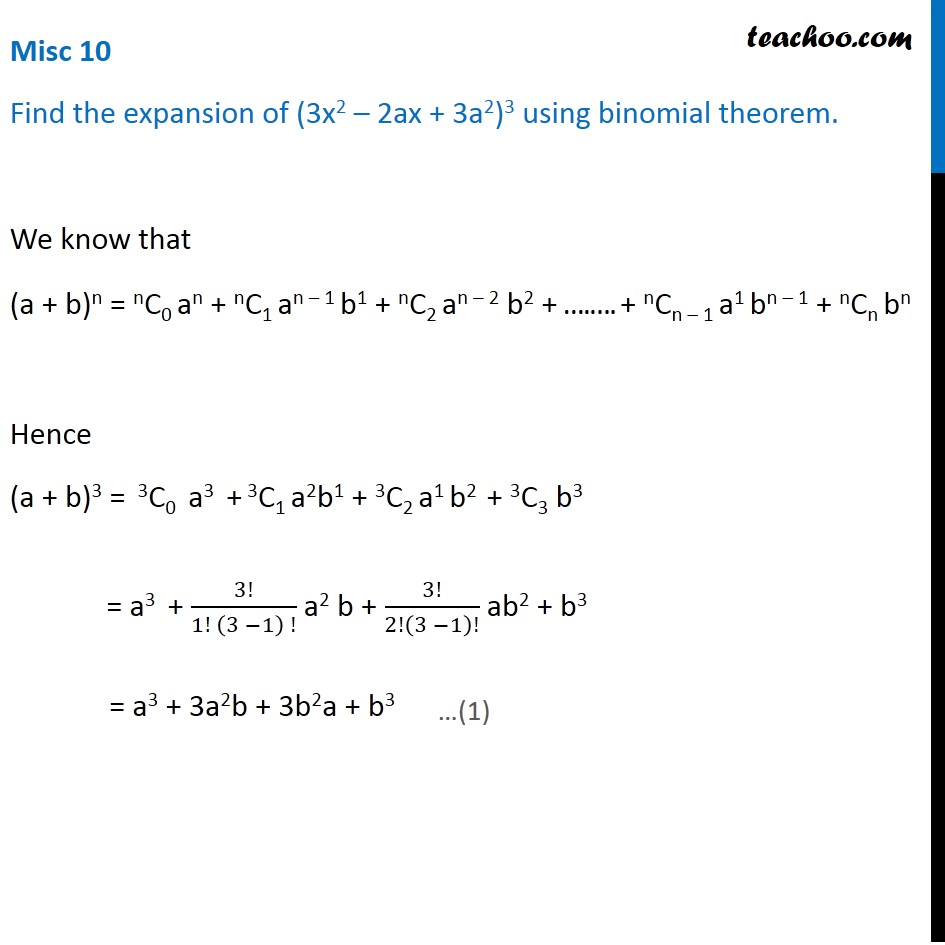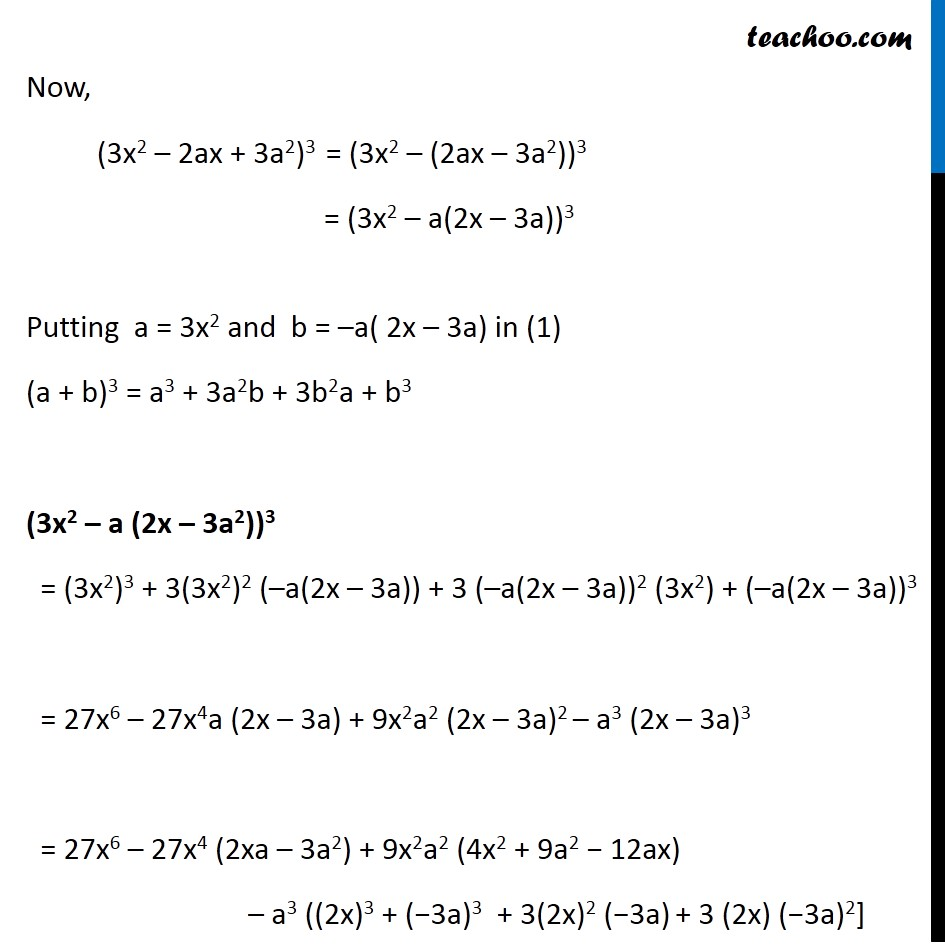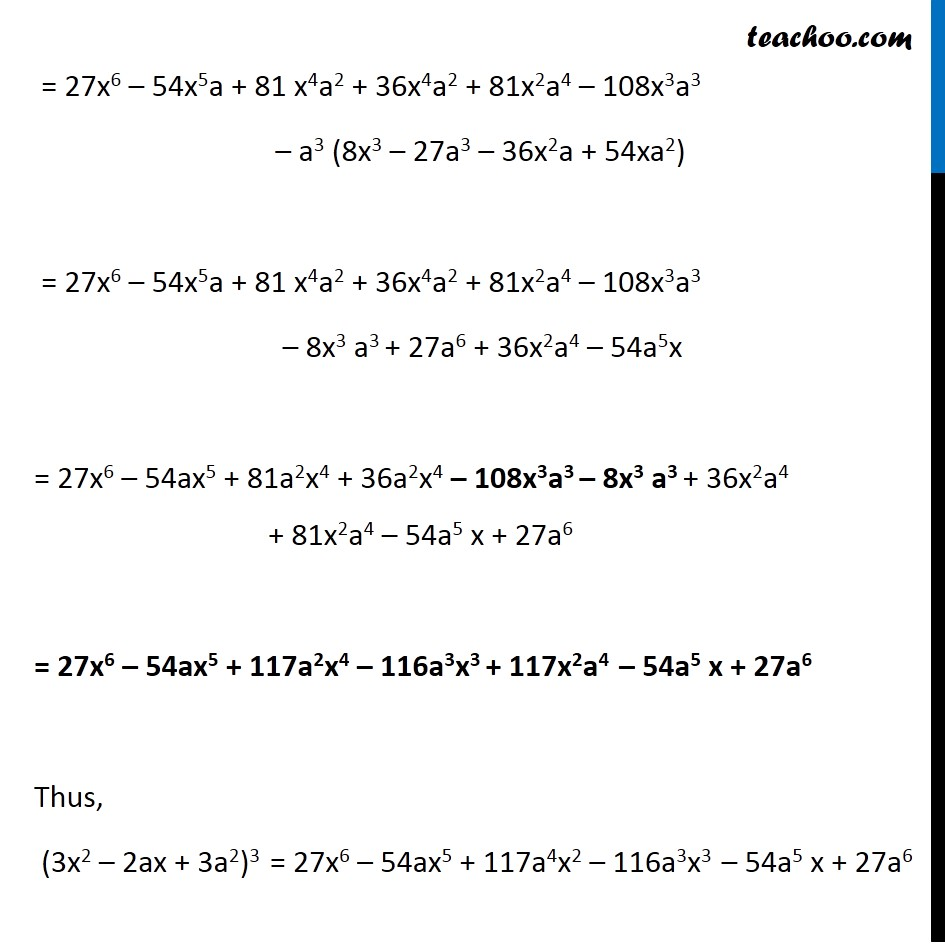1. Chapter 8 Class 11 Binomial Theorem (Deleted)
2. Serial order wise
3. Miscellaneous

Transcript

Misc 10 Find the expansion of (3x2 – 2ax + 3a2)3 using binomial theorem. We know that (a + b)n = nC0 an + nC1 an – 1 b1 + nC2 an – 2 b2 + ….…. + nCn – 1 a1 bn – 1 + nCn bn Hence (a + b)3 = 3C0 a3 + 3C1 a2b1 + 3C2 a1 b2 + 3C3 b3 = a3 + 3!/(1! (3 −1) !) a2 b + 3!/2!(3 −1)! ab2 + b3 = a3 + 3a2b + 3b2a + b3 Now, (3x2 – 2ax + 3a2)3 = (3x2 – (2ax – 3a2))3 = (3x2 – a(2x – 3a))3 Putting a = 3x2 and b = –a( 2x – 3a) in (1) (a + b)3 = a3 + 3a2b + 3b2a + b3 (3x2 – a (2x – 3a2))3 = (3x2)3 + 3(3x2)2 (–a(2x – 3a)) + 3 (–a(2x – 3a))2 (3x2) + (–a(2x – 3a))3 = 27x6 – 27x4a (2x – 3a) + 9x2a2 (2x – 3a)2 – a3 (2x – 3a)3 = 27x6 – 27x4 (2xa – 3a2) + 9x2a2 (4x2 + 9a2 − 12ax) – a3 ((2x)3 + (−3a)3 + 3(2x)2 (−3a) + 3 (2x) (−3a)2] = 27x6 – 54x5a + 81 x4a2 + 36x4a2 + 81x2a4 – 108x3a3 – a3 (8x3 – 27a3 – 36x2a + 54xa2) = 27x6 – 54x5a + 81 x4a2 + 36x4a2 + 81x2a4 – 108x3a3 – 8x3 a3 + 27a6 + 36x2a4 – 54a5x = 27x6 – 54ax5 + 81a2x4 + 36a2x4 – 108x3a3 – 8x3 a3 + 36x2a4 + 81x2a4 – 54a5 x + 27a6 = 27x6 – 54ax5 + 117a2x4 – 116a3x3 + 117x2a4 – 54a5 x + 27a6 Thus, (3x2 – 2ax + 3a2)3 = 27x6 – 54ax5 + 117a4x2 – 116a3x3 – 54a5 x + 27a6

Miscellaneous